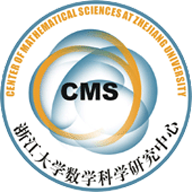(13002)CURVATURES OF DIRECT IMAGE SHEAVES OF VECTOR BUNDLES　AND APPLICATIONS

Abstract. Let p : X ! S be a smooth K¨ahler fibration and E ! X a Hermitian holomorphic vector bundle. As motivated by the work of Berdtsson([Bern09]), by using basic Hodge theory, we derive several general curvature formulas for the direct image p(KX/S E) for general Hermitian holomorphic vector bundle E in a very simple way. A straightforward
application is that, if the Hermitian vector bundle E is Nakano-negative along the base S, then the direct image p(KX/S  E) is Nakano-negative. We also use these curvature formulas to study the moduli space of projectively flat vector bundles with positive first Chern classes and obtain that, if the Chern curvature of direct image p(KX
E)–of a positive projectively flat family (E, h(t))t2D ! X–vanishes, then the curvature forms of this family are connected by holomorphic automorphisms of the pair (X,E).

• 1303.2701.pdf0.pdf